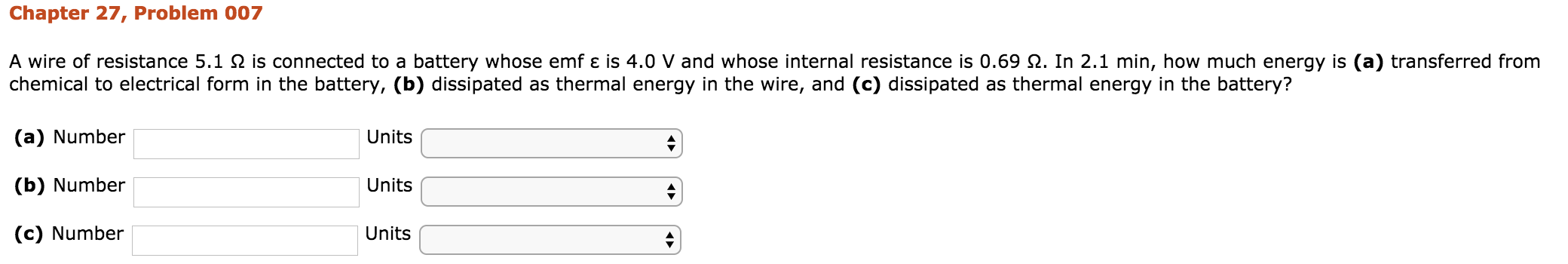# Chapter 27, Problem 007fromA wire of resistance 5.1 Ω is connected to a battery whose emf ε is 4.0 V and whose internal resistance is 0.69 Ω. In 2.1 min, how much energy is (a) transferred fromeergy In the narerg s()chemical to electrical form in the battery, (b) dissipated as thermal energy in the wire, and (c) dissipated as thermal energy in the battery?(a) Number(b) Number(c) NumberUnitsUnitsUnits

Question
3 viewshelp_outlineImage TranscriptioncloseChapter 27, Problem 007 from A wire of resistance 5.1 Ω is connected to a battery whose emf ε is 4.0 V and whose internal resistance is 0.69 Ω. In 2.1 min, how much energy is (a) transferred from eergy In the narerg s() chemical to electrical form in the battery, (b) dissipated as thermal energy in the wire, and (c) dissipated as thermal energy in the battery? (a) Number (b) Number (c) Number Units Units Units fullscreen
check_circle

Step 1

Given: R = 5.1 ohm

r = 0.69 ohm

emf = 4 V

t = 2.1 min = 2.1 × 60 = 126 seconds

Find: (a) The energy transferred from chemical to electrical form in the battery.

(b) The energy dissipated as thermal energy in the wire.

(c) The energy disspated as thermal energy in the battery.

Step 2

(a) Calculate the energy transferred from chemical to electrical form in the battery as:

Energy = power × time

Step 3

(b) Calculate the energy disspated as thermal energy in the wire:

...

### Want to see the full answer?

See Solution

#### Want to see this answer and more?

Solutions are written by subject experts who are available 24/7. Questions are typically answered within 1 hour.*

See Solution
*Response times may vary by subject and question.
Tagged in

### Current Electricity